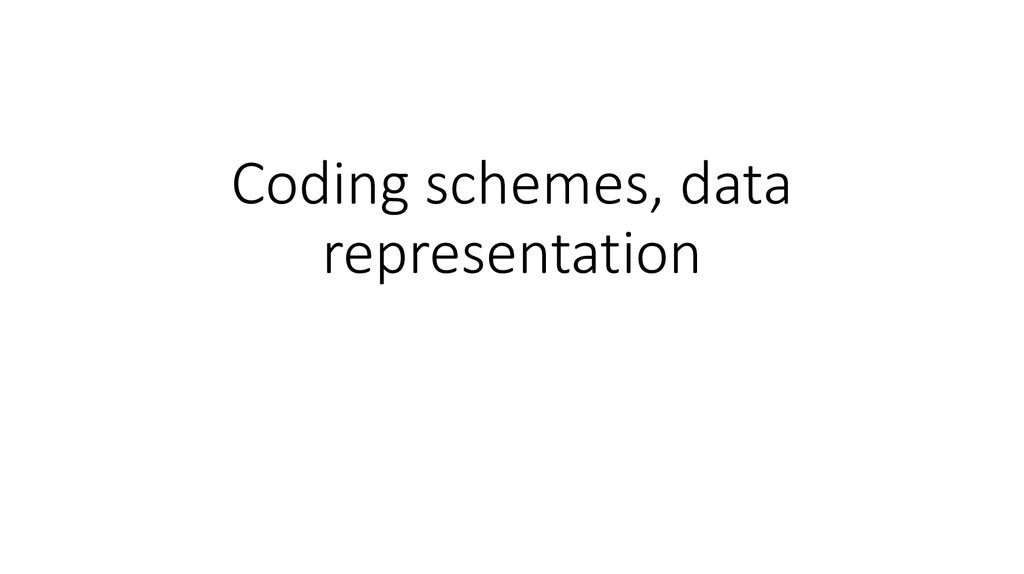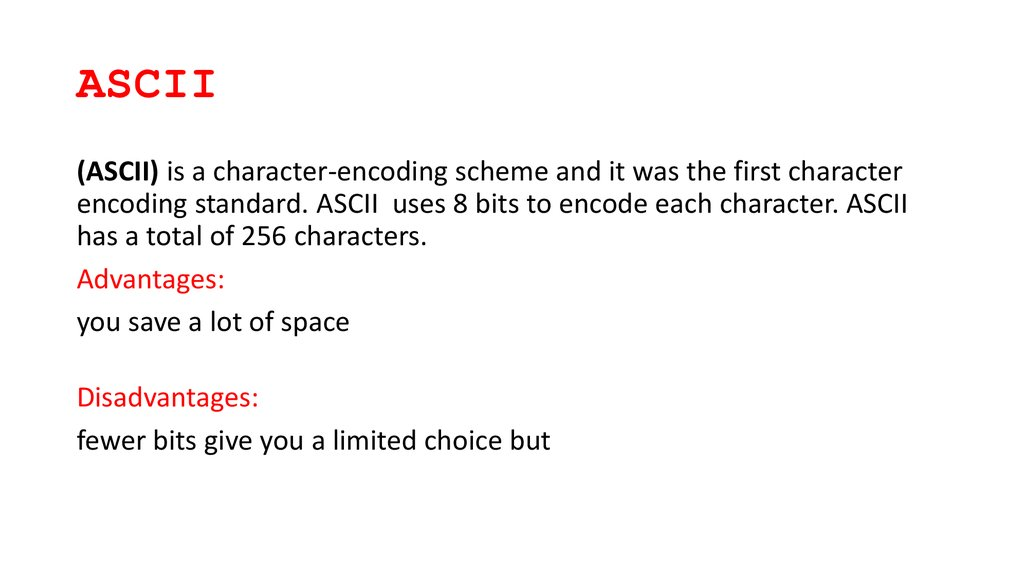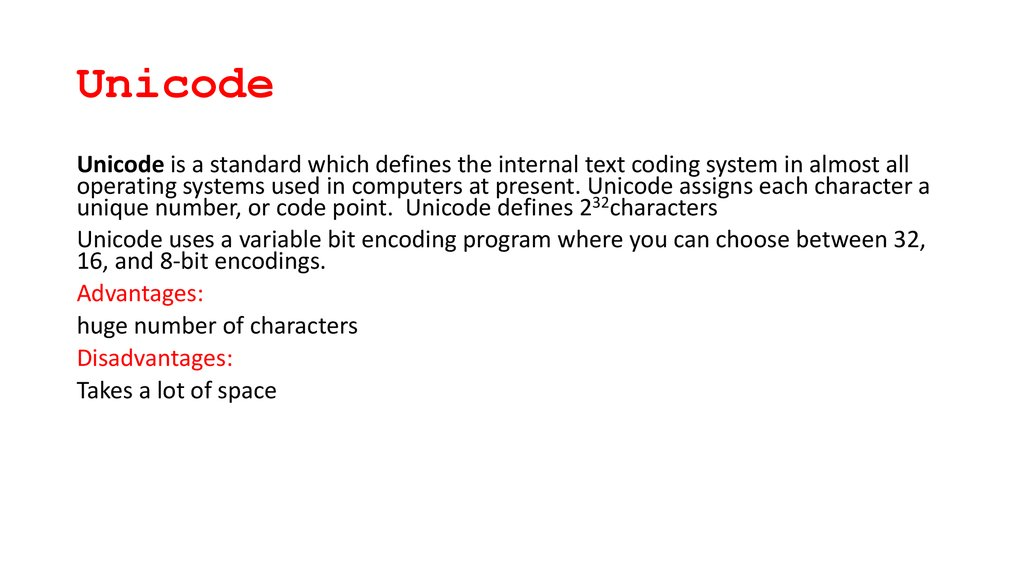# Coding schemes, data representation

## 1.

Coding schemes, data
representation

## 2. ASCII

(ASCII) is a character-encoding scheme and it was the first character
encoding standard. ASCII uses 8 bits to encode each character. ASCII
has a total of 256 characters.
you save a lot of space
fewer bits give you a limited choice but

## 3. Unicode

Unicode is a standard which defines the internal text coding system in almost all
operating systems used in computers at present. Unicode assigns each character a
unique number, or code point. Unicode defines 232characters
Unicode uses a variable bit encoding program where you can choose between 32,
16, and 8-bit encodings.
huge number of characters
Takes a lot of space

## 4. Summary

1.ASCII uses an 8-bit encoding while Unicode uses a variable bit
encoding.
2.Unicode is standardized while ASCII isn’t.
3.Unicode represents most written languages in the world while ASCII
does not.
4.ASCII has its equivalent within Unicode.

## 6. Why we use different types of data?

Data types are blocks or limited area confined for storing some specific
item. Data type of int type can store integer value. In the same way
there are many other data type double, float, char which can store
large integer value, large decimal value, and character value.

## 7.

Fixed Point and Floating Point
Number Representations

## 8. Signed binary numbers

• 0000 0101 (positive)
• 1111 1011 (negative)

## 13.

Example: denary to binary floating point
If we are asked to convert the denary number 39.75 into binary floating point we first need to find out
the binary equivalent:
128 64 32 16 8 4 2 1 . ½ ¼ ⅛
0
0 1 0 0 1 1 1 . 1 1 0
How far do we need to move the binary point to the left so that the number is normlised?
0 0 . 1 0 0 1 1 1 1 1 0 (6 places to the left)
So to get our decimal point back to where it started, we need to move 6 places to the right. 6 now